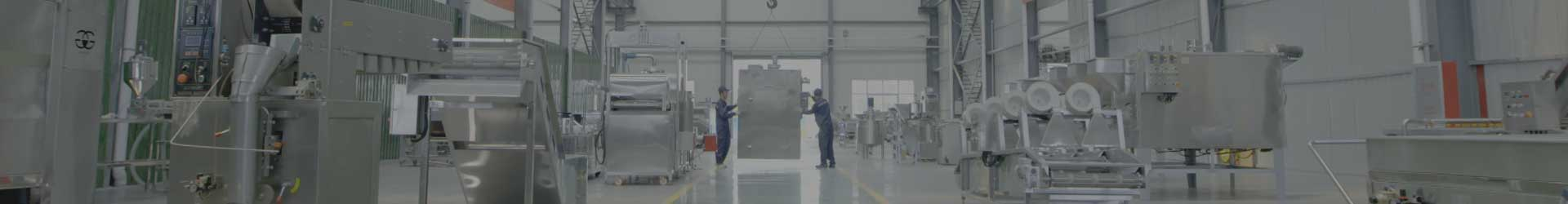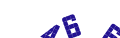+86 18595613260## News

We will update our company dynamics and technical information in time.

# Heat Pump Energy Analysis

2023-01-30

In the heat pump cycle, the heat of Q0 kcal/h is obtained from the low-temperature heat source (outdoor air or circulating water, whose temperature is higher than the evaporation temperature t0), and the mechanical work ALkcal/h is consumed, which is supplied to the high-temperature heat source (indoor heating system) Q1 kcal/h heat, the relationship between these heat is in line with the first law of thermodynamics, that is, Q1 =Q0+AL kcal/h.

If the heat pump device is not used, but the heat converted by mechanical work (or the high-temperature heat source is directly heated by electric energy, the heat obtained is ALkcal/h, and after the heat pump device is used, the high-temperature heat source (heating system) obtains more heat: Q1-AL=Q0 kcal/h. This heat is obtained from a low-temperature heat source. If the heat pump device is not used, this heat cannot be obtained. Therefore, the heat pump device can save fuel and use waste heat.

The working cycle of a heat pump is exactly the opposite of that of a heat engine. A heat engine uses the energy of a high-temperature heat source to generate mechanical work, while a heat pump transfers heat from a low-temperature heat source to a high-temperature object by consuming mechanical work.

If the heat pump and the heat engine have the same heat source temperature, the thermal efficiency of the heat engine cycle η=AL/Q1; the energy index of the heat engine cycle - - - - heat conversion coefficient φ=Q1/AL, so φ=1/η. The value of η is always less than 1, so the value of p is greater than 1.

If the refrigerator and the heat pump have two identical heat source temperatures, the relationship between them is: φ=Q1/AL= (Q0+AL)/AL=ε+1, where ε is the refrigeration coefficient of the refrigerator. It can be seen from this that the minimum value of the heat conversion coefficient is p=1, in this limit case E=0, Q0=0, that is, no heat is absorbed from the low-temperature heat source.